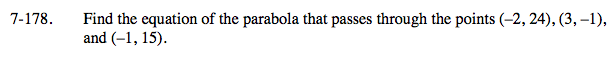Home > A2C > Chapter 7 > Lesson 7.3.2 > Problem7-178

7-178.

Find the equation of the parabola that passes through the points (−2, 24), (3, −1), and (−1, 15). Homework Help ✎Substitue the points into the general equation y = ax2 + bx + c.

Use elimination to solve the system of equations.

Substitute the values you found for a, b, and c into the general equation from the first hint.